# 5 Minute Check on Chapter 2 Transparency 3

• Slides: 145 -Minute Check on Chapter 2 Transparency 3 -1 1. Evaluate 42 - |x - 7| if x = -3 2. Find 4. 1 (-0. 5) Simplify each expression 3. 8(-2 c + 5) + 9 c 4. (36 d – 18) / (-9) 5. A bag of lollipops has 10 red, 15 green, and 15 yellow lollipops. If one is chosen at random, what is the probability that it is not green? 6. Standardized Test Practice: A 8/4 < 4/8 B Which of the following is a true statement -4/8 < -8/4 C -4/8 > -8/4 Click the mouse button or press the Space Bar to display the answers. D -4/8 > 4/8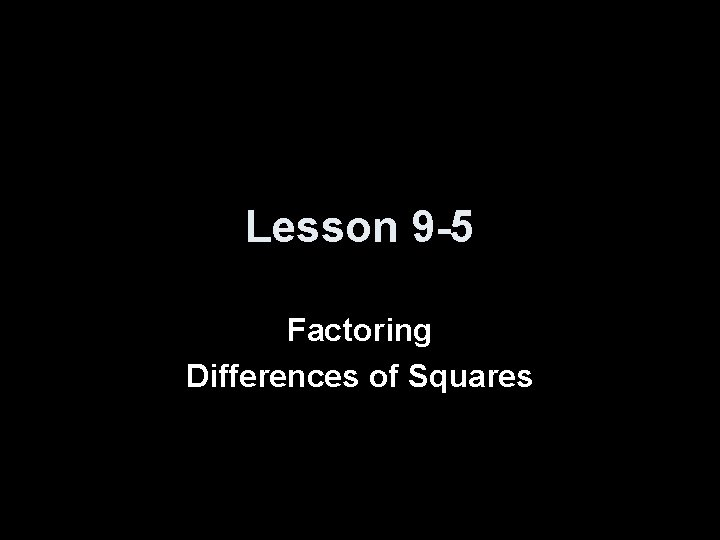Lesson 9 -5 Factoring Differences of Squares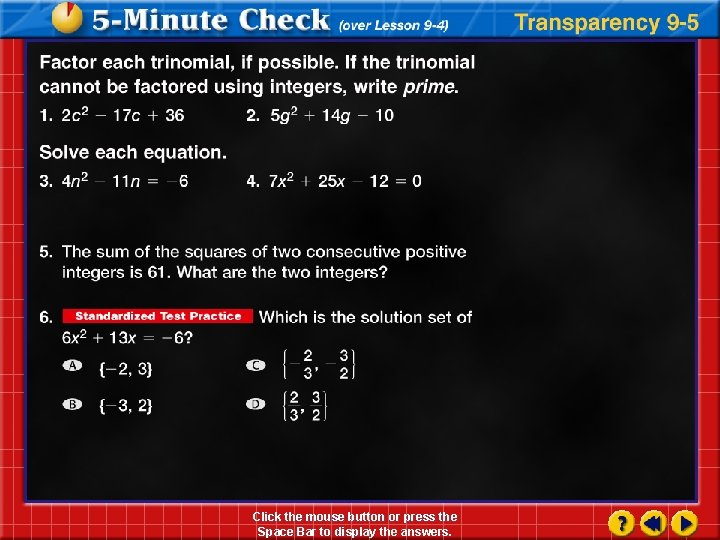Click the mouse button or press the Space Bar to display the answers.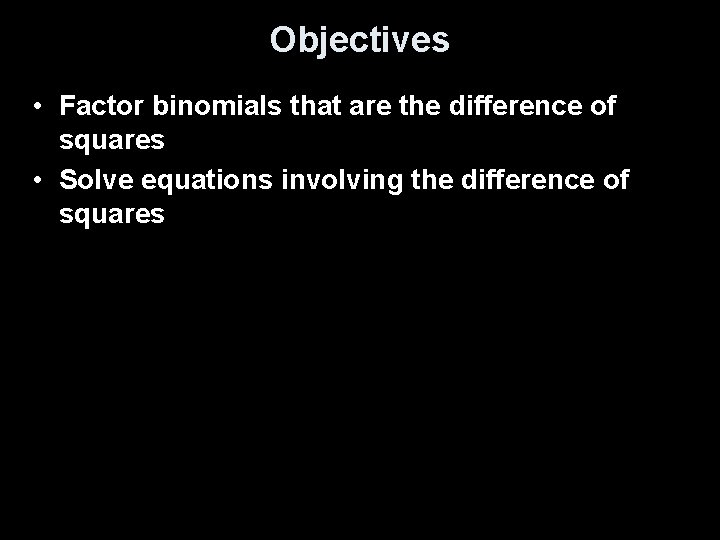Objectives • Factor binomials that are the difference of squares • Solve equations involving the difference of squaresVocabulary • NA –Difference of Squares • Symbols: a 2 – b 2 = (a + b) (a – b) or (a – b) (a + b) • Examples: – x 2 – 9 = (x – 3) (x + 3) or (x + 3) (x – 3) – b 2 – 25 = (b – 5) (b + 5) or (b + 5) (b – 5)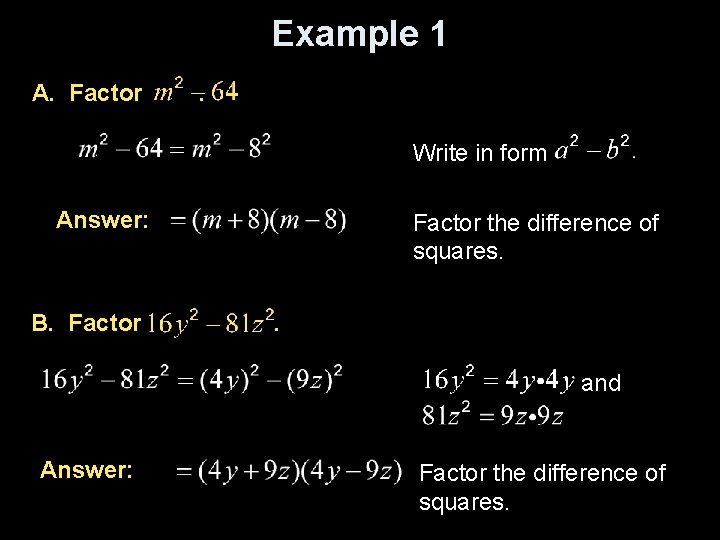Example 1 A. Factor . Write in form Answer: B. Factor the difference of squares. . and Answer: Factor the difference of squares.Example 2 Factor The GCF of and 27 b is 3 b. and Answer: Factor the difference of squares.Example 3 Factor The GCF of and 2500 is 4. and Factor the difference of squares. and Answer: Factor the difference of squares.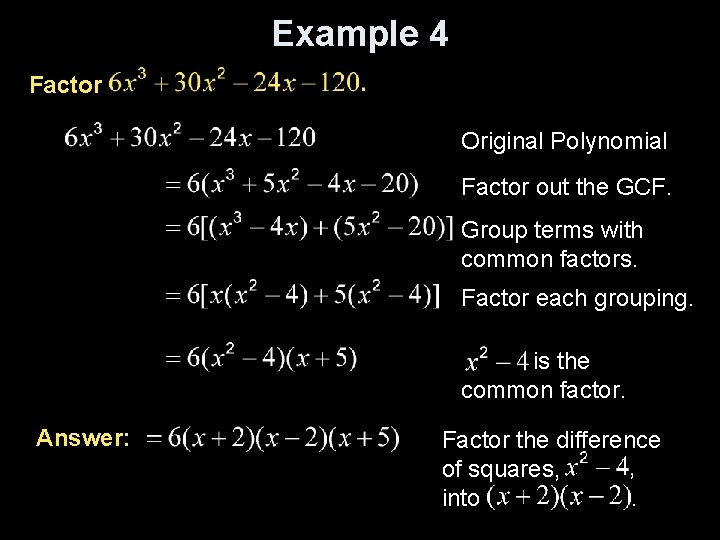Example 4 Factor Original Polynomial Factor out the GCF. Group terms with common factors. Factor each grouping. is the common factor. Answer: Factor the difference of squares, into.Example 5 a A. Solve by factoring. Original equation. and Factor the difference of squares. or Zero Product Property Solve each equation. Answer: The solution set is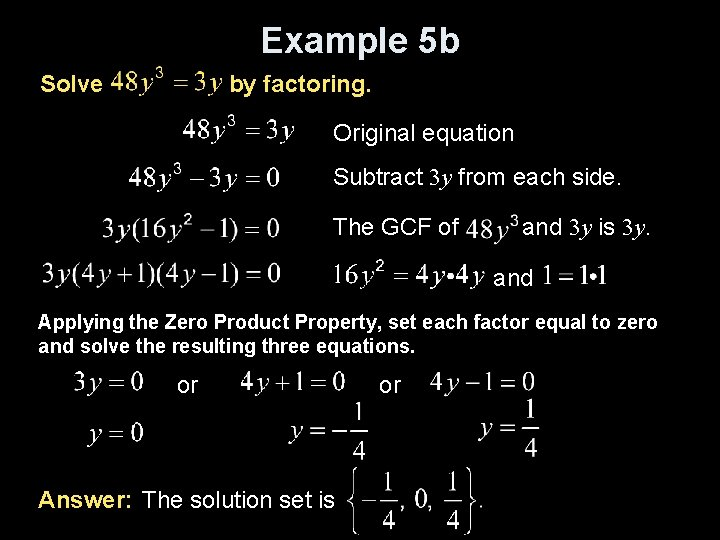Example 5 b Solve by factoring. Original equation Subtract 3 y from each side. The GCF of and 3 y is 3 y. and Applying the Zero Product Property, set each factor equal to zero and solve the resulting three equations. or Answer: The solution set is or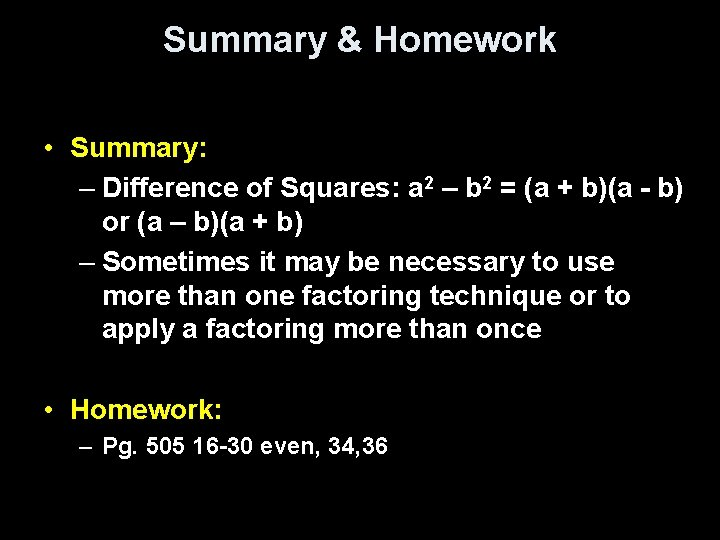Summary & Homework • Summary: – Difference of Squares: a 2 – b 2 = (a + b)(a - b) or (a – b)(a + b) – Sometimes it may be necessary to use more than one factoring technique or to apply a factoring more than once • Homework: – Pg. 505 16 -30 even, 34, 36